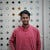# Computational Complexity of PCA

If you know the basics PCA you probably know that PCA is computationally extensive. This article gives an intuition behind all the extensive mathematical calculation behind PCA. Let’s dive deep.

A simple way of computing PCA of a matrix X is to compute the eigenvalue decomposition of its covariance matrix. Given N ×D matrix X, a target dimensionality d, the algorithm computes an N ×d matrix V such that the columns of V are the principal components of X.

The algorithm for computing the eigenvalue decomposition of the covariance matrix can be summarized in the following steps:

# Interview Questions on PCA

Here’s a bunch of Interview Questions asked on Principal Component Analysis

1. Explain the Curse of Dimensionality?

The curse of dimensionality refers to all the problems that arise working with data in the higher dimensions. As the number of features increase, the number of samples increases, hence, the model becomes more complex. The more the number of features, the more the chances of overfitting. A machine learning model that is trained on a large number of features, gets increasingly dependent on the data it was trained on and in turn overfitted, resulting in poor performance on real data, beating the purpose…

# Computational Complexity of SVM

If you are pursuing Computer Science, probably you must have come across the notations of time complexity.

There’s not a single technical interview round that’s not going to question you on running time complexity of an algorithm.

The time complexity of an algorithm is the total amount of time required by an algorithm to complete its execution. In simple words, every piece of code we write takes time to execute. The time taken by any piece of code to run is known as the time complexity of that code. The lesser the time complexity, the faster the execution.

WHY SHOULD…

# Interview Questions on SVM

Support Vector Machine” (SVM) is a supervised machine learning algorithm which can be used for both classification or regression problems.

SVMs are particularly well suited for classification of complex but small- or medium-sized datasets.

We’ll talk about some Interview questions related to SVMs.

1. Explain SVM to a non-technical person.

Explanation: Suppose you have to construct a bidirectional road. Now you have to make a dividing line. The optimal approach would be to make margins on the sides and draw an equidistant line from both the margins.

# Linear Regression from Scratch

Linear Regression needs no introduction. It’s the ‘Hello World’ of Machine Learning World. Let’s deep dive into the use cases of Linear Regression and mathematical intuition behind it.

We are in a world driven by data. As commonly said, ‘Data is the new oil’. One of the most important types of data analysis is regression. Regression aims to build a mathematical model that can be used to predict the values of a dependent variable based upon the values of an independent variable. …

# Importance of Distance Metrics in Machine Learning

You read the title right !!! You might be wondering how ‘Distance’ and ‘Machine Learning’ are related? Am I going out of context?

No, if you have hands-on experience with Machine Learning algorithms, undoubtedly you came across ‘Distance’ as a parameter. This blog focuses on the need for distance metrics in Machine learning and it’s use cases so that you appreciate the concept more.

# Distance Metrics

The metric is a function that defines a concept of distance between any two members of the set, which are usually called points.

The metric satisfies a few simple properties.

## * Non-negativity:

d(i,j)≥0: Distance is a non-negative number.

# Role of Mathematics in Machine Learning

Nowadays many people are planning the transition to AI/ML/Data Science world which is very encouraging and matching the pace of changing the world.

But these people are confused with questions like :

Various problems are hard to solve using traditional…

# Understanding Different Job Roles in the field of Data Science